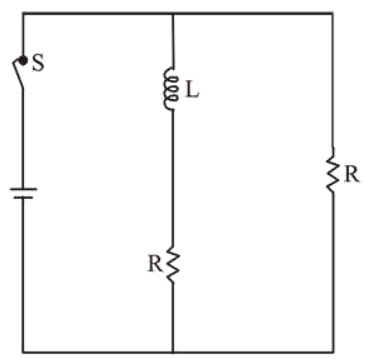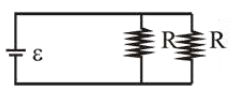# In the figure shown, a circuit contains two identical resistors with resistance

Question:

In the figure shown, a circuit contains two identical resistors with resistance $\mathrm{R}=5 \Omega$ and an inductance with $\mathrm{L}=2 \mathrm{mH}$. An ideal battery of $15 \mathrm{~V}$ is connected in the circuit. What will be the current through the battery long after the switch is closed?1. (1) $5.5 \mathrm{~A}$

2. (2) $7.5 \mathrm{~A}$

3. (3) $3 \mathrm{~A}$

4. (4) $6 \mathrm{~A}$

Correct Option: , 4

Solution:

(4)]

Long time after switch is closed, the inductor will be idle so, the equivalent diagram will be as below$I=\frac{\varepsilon}{\left(\frac{R \times R}{R+R}\right)}=\frac{2 \varepsilon}{R}=\frac{2 \times 15}{5}=6 \mathrm{~A}$